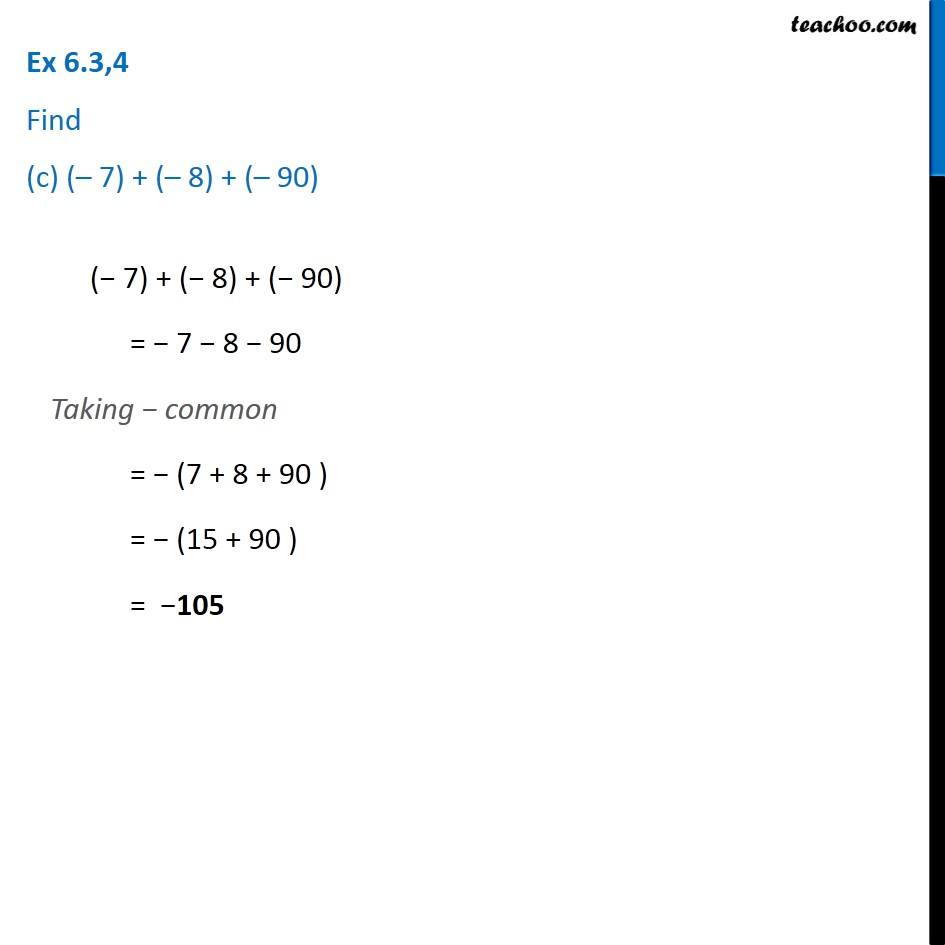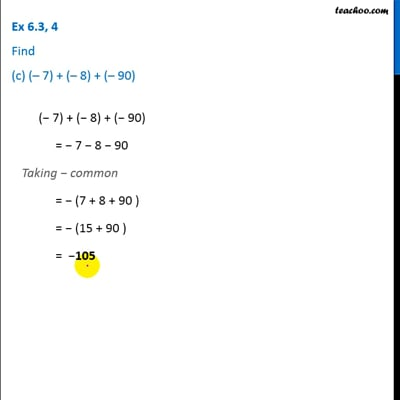Ex 6.3

Chapter 6 Class 6 Integers
Serial order wiseThis video is only available for Teachoo black users

Introducing your new favourite teacher - Teachoo Black, at only ₹83 per month

### Transcript

Ex 6.3,4 Find (c) (– 7) + (– 8) + (– 90)(− 7) + (− 8) + (− 90) = − 7 − 8 − 90 Taking − common = − (7 + 8 + 90 ) = − (15 + 90 ) = −105